# Cones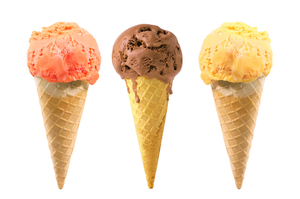Many things, like party hats and ice cream cones are shaped like cones. Wizard's hats and dunce caps are also cone-shaped. A witch's hat is shaped a bit like a cone with a brim.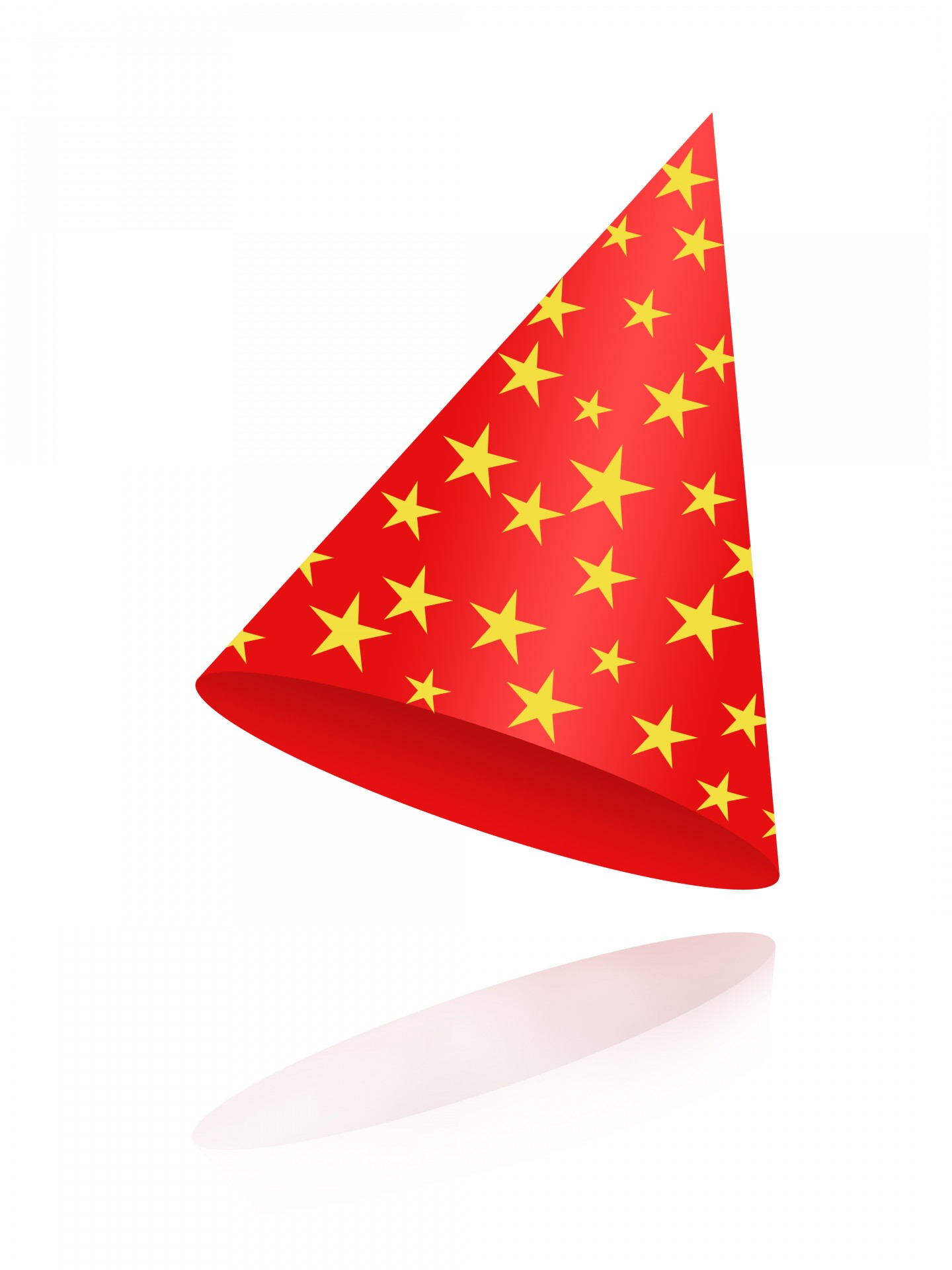We see traffic cones on our roads. They have a shape like a cone, with a bit sawn off the top and a widened-out base so that they don't topple over. We call objects that are shaped like cones conical. So, what exactly is a cone, and what are its properties?

## The Structure of a Cone

Cones are solids with a flat base, and one curved side. The flat base may be shaped like a circle or an ellipse. Most of the time, it's a circle. Cones are not polyhedrons as they have a curved surface, and no straight edges.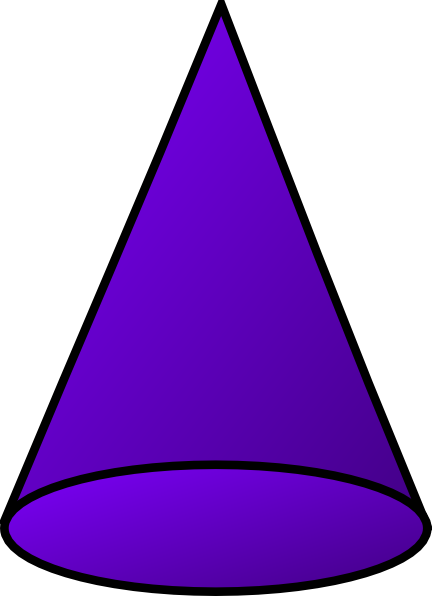The flat bottom of a cone is called its base, and the pointy bit at the top of the cone is called its apex, as shown in the picture below.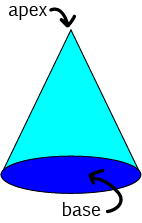## How Do Mathematicians Build a (Circular) Cone?

You may have made a cone yourself at some stage, by taking a bit of cardboard shaped like the one shown below and bending it around until the edges meet.I've made them as witches and wizards hats, and as lolly baskets for the Christmas tree.

Mathematicians have a slightly more abstract view of how to create a cone. They take a right-angled triangle and rotate it around one of its two shorter sides: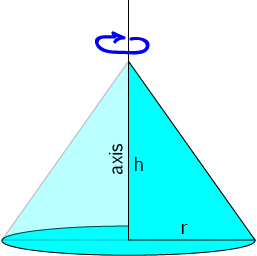The side that the triangle is rotated around is called the axis of the cone.

## Right and Oblique Cones

Just as with pyramids, there are two types of cones: right cones are cones in which the apex lies directly about the centre of the base, and oblique cones are cones in which it doesn't: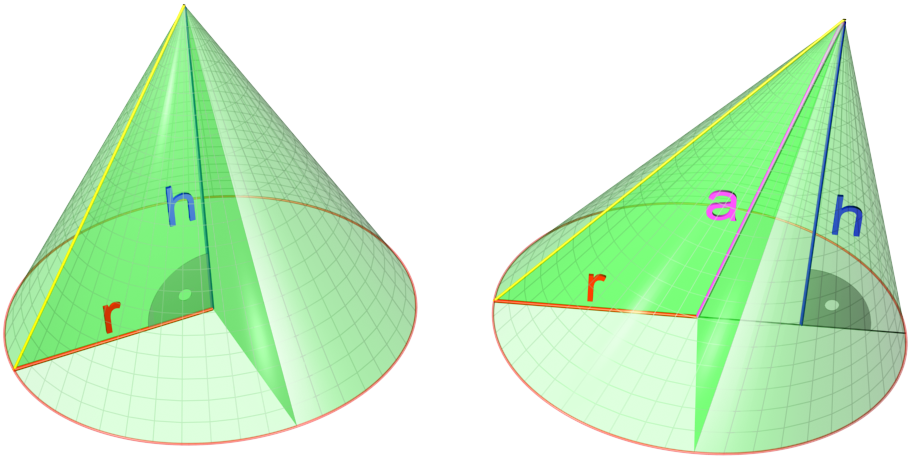In the above picture, the cone on the left is a right cone, while the cone on the right is an oblique cone.

## Finding the Surface Area of a Cone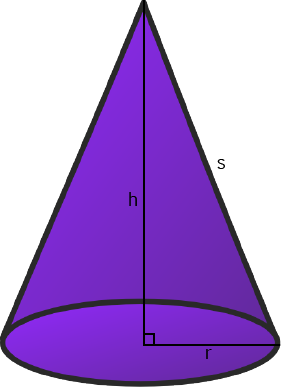The surface area of a cone may be split up into two parts:

• The area of the base. For a circular cone, this is $\pi r^2$, where $r$ is the radius of the cone.
• The area of the curved surface. This is equal to $\pi r s$, where $r$ is the radius of the cone, and $s$ is the slant height of the cone as shown in the picture on the left.
Adding these two quantities together gives the surface area of the cone:
$SA =\pi r^2 + \pi rs = \pi r(r + s)$.

Note that we can use Pythagoras' theorem to find $s = \sqrt{r^2 + h^2}$.

For example, if we were asked to find the surface area of a cone with height $h = 3$ cm and radius $r = 4$ cm, then we would calculate $s = \sqrt{3^2 + 4^2} = 5$ cm, and

$SA = \pi (4)(4 + 3) = 28 \pi \approx 88 \text{ cm}^2$.

## Finding the Volume of a Cone

The formula for the volume of a right circular cone is

$V = \dfrac{1}{3} \pi r^2 h$,
where $h$ is the height of the cone, and $r$ is the radius of its base.

For example, the volume of a cone with height $h = 3$ cm and base radius $r = 4$ cm is given by

$V = \dfrac{1}{3} \pi (4)^2(3) = 16 \pi \approx 50.3 \text{ cm}^3$.

### Volumes of Cones and Volumes of Cylinders

Did you notice something about the formula for the volume of a cone? It looks a lot like the formula for the volume of a cylinder.

In fact, we can draw a cylinder of the same base radius and height around a cone, as shown in the picture below:The formula for the volume of the cylinder is $V = \pi r^2 h$, so the volume of the cone is equal to $\dfrac{1}{3}$ of the volume of the cylinder of the same base radius and height.

If $\dfrac{1}{3}$ is sounding awfully familiar to you, that's because the volume of a right pyramid is also equal to $\dfrac{1}{3}$ of the volume of the prism of the same base and height. This isn't a coincidence: you can think of a cone as a "pyramid with a circular base".

### Description

There are several lessons related to 3D geometry such as

1. Euler's formula
2. Vertices, Edges and Faces
3. Volumes of 3D shapes
4. etc

Even though we've titled this lesson series to be more inclined for Year 10 or higher students, however, these lessons can be read and utilized by lower grades students.

### Prerequisites

Understanding of 3D shapes

### Audience

Year 10 or higher, but suitable for Year 8+ students

### Learning Objectives

Get to know 3D Geometry

Author: Subject Coach
You must be logged in as Student to ask a Question.